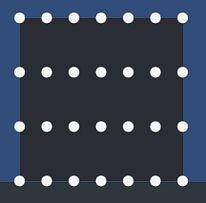##### Honours Project

My honours project was titled Evaluating the Benefits of Finite Element Analysis in the Simulation of the Deformation of Solid Objects in Real time. It was an extensive look into the use of Finite Element Analysis (FEA) for structural analysis in the auto motive industry.

The result of the project was a 2D shape within Unity that went slightly beyond the physics already built in with Box2D to allow for simple deformable shapes. The quadrilateral shape could be resized and the nodes that created the structure within could be distributed evenly across the structure in various degrees of detail. All of this went towards ensuring the shape deformed from collisions in a uniform manner.

To create a working FEA system, the process was broken down into several steps:

• Discretisation - The shape was needed to be broken up into a set of quadrilateral elements at runtime. This was done based on the series of nodes that were spread across the shape.

• Element properties - Each element needed certain properties worked out at run time to build the stiffness matrix that the FEA system depended on for calculations. This meant first the stress-displacement matrix and the elasticity matrix were needed.

• Collision - At the point of a collision, it was needed for the incoming force and point of contact to be found. The force of the collision and the position of the collision in world space was provided by Unity, the system needed to be able to calculate which node on the shape was the closest to the collision.

• Force Propagation - Once the point of the shape of the collision was found and the force of the impact was found, the system was then required to work out how far the force would propagate into the shape and to what degree. This was achieved by using the inital node and applying a lesser force through to the neighbouring nodes and repeating the process for several layers of nodes until the force is negligible.

• Deformation - Once the force has been applied to the relevant nodes, the system uses resultant force and stiffness matrix for each node to calculate a global displacement matrix used for every element in the shape. Once the displacement for each element has been applied, the mesh for each element is recalculated to show the visual result of the deformation.

## How the discretisation was achieved